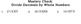# Divide Decimals by Whole Numbers, 5/6th grade - worksheets - Individualized MathSubject
Resource Type
File Type

PDF

(1020 KB|9 pages)
Standards
• Product Description
• StandardsNEW

A set of 6 worksheets and a quiz on dividing decimals by whole numbers, designed for fifth and sixth grade level. Begins with placing the decimal point above the one in the dividend, teaches zero as a placeholder in decimals, and to extend the dividend with zeros in order to continue dividing until there is no remainder from subtracting.

Can be used in tutoring or as a resource with specific students who need more focused practice on the concept. May be used to quickly review concept or help student re-learn it. I recommend using different pages on different days so the student has to move information in and out of memory more.

I created this to use with my tutoring students; if they're having difficulty with dividing a decimal number, these worksheets always give them the practice they need to become successful with the concept!

This set of worksheets was created by Destiny Woods. You can find more Individualized Math worksheets here. To see all my other products, visit my store.

Fluently add, subtract, multiply, and divide multi-digit decimals using the standard algorithm for each operation.
Add, subtract, multiply, and divide decimals to hundredths, using concrete models or drawings and strategies based on place value, properties of operations, and/or the relationship between addition and subtraction; relate the strategy to a written method and explain the reasoning used.
Total Pages
9 pages
Not Included
Teaching Duration
N/A
Report this Resource to TpT
Reported resources will be reviewed by our team. Report this resource to let us know if this resource violates TpT’s content guidelines.